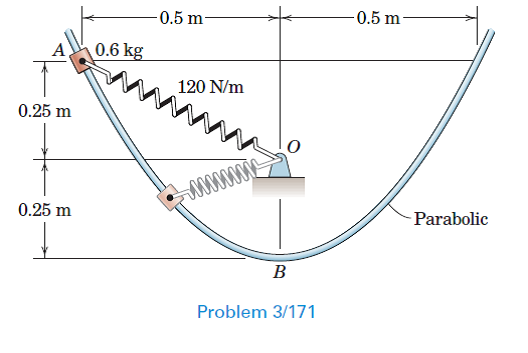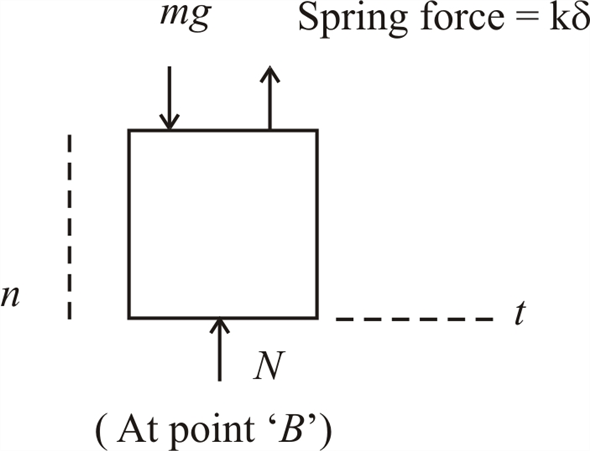Problem

# The 0.6-kg slider is released from rest at A and slides down the smooth parabolic guide...

The 0.6-kg slider is released from rest at A and slides down the smooth parabolic guide (which lies in a vertical plane) under the influence of its own weight and of the spring of constant 120 N /m. Determine the speed of the slider as it passes point B and the corresponding normal force exerted on it by the guide. The unstretched length of the spring is 200 mm.#### Step-by-Step Solution

Solution 1

Given that,

Mass of the slider, $$m=0.6 \mathrm{~kg}$$

Stiffness of the spring, $$k=120 \mathrm{~N} / \mathrm{m}$$

Unstretched length of the spring $$=200 \mathrm{~mm}$$

Initial velocity of the slider at $$A$$ is $$v_{A}=0$$

Velocity of the slider at $$B$$ is $$v_{B}$$

Initial deflection of the spring at $$A, x_{A}=\sqrt{0.25^{2}+0.5^{2}}-0.2$$ $$x_{A}=0.359 \mathrm{~m}$$

Deflection of the spring at $$B, x_{B}=0.25-0.2$$

$$x_{B}=0.05 \mathrm{~m}$$

Taking datum at ' $$B$$ '

\begin{aligned} &T_{A}+V_{A}=T_{B}+V_{B} \\ &0+0.6(9.81)(0.5)+\frac{1}{2} \times 120 \times 0.359^{2}=\frac{1}{2}(0.6) v_{B}^{2}+\frac{1}{2}(120)[0.05]^{2} \\ &2.943+7.733=0.3 v_{B}^{2}+0.15 \\ &v_{B}=5.923 \mathrm{~m} / \mathrm{s} \end{aligned}

Equation of the parabola, $$y=k x^{2}$$

From the given problem, at point $$B: 0.5=k(0.5)^{2}$$ $$k=2$$

Then equation of parabola is $$y=2 x^{2}$$

\begin{aligned} &\frac{d y}{d x}=4 x \\ &\frac{d^{2} y}{d x^{2}}=4 \end{aligned}

Radius of curvature, $$\rho=\frac{\left[1+\left(\frac{d y}{d x}\right)^{2}\right]^{\frac{3}{2}}}{\frac{d^{2} y}{d x^{2}}}$$

When $$x=0$$

\begin{aligned} &\rho=\frac{\left[1+0^{2}\right]^{\frac{3}{2}}}{4} \\ &=0.25 \mathrm{~m} \end{aligned}Let $$N$$ be the normal force exerted by the guide

\begin{aligned} &\sum F_{n}=m a_{n} \\ &N+k x_{B}-m g=m \frac{v_{B}^{2}}{\rho} \\ &N+120(0.05)-0.6(9.81)=0.6 \times \frac{5.923^{2}}{0.25} \\ &N=84.08 \mathrm{~N} \end{aligned}